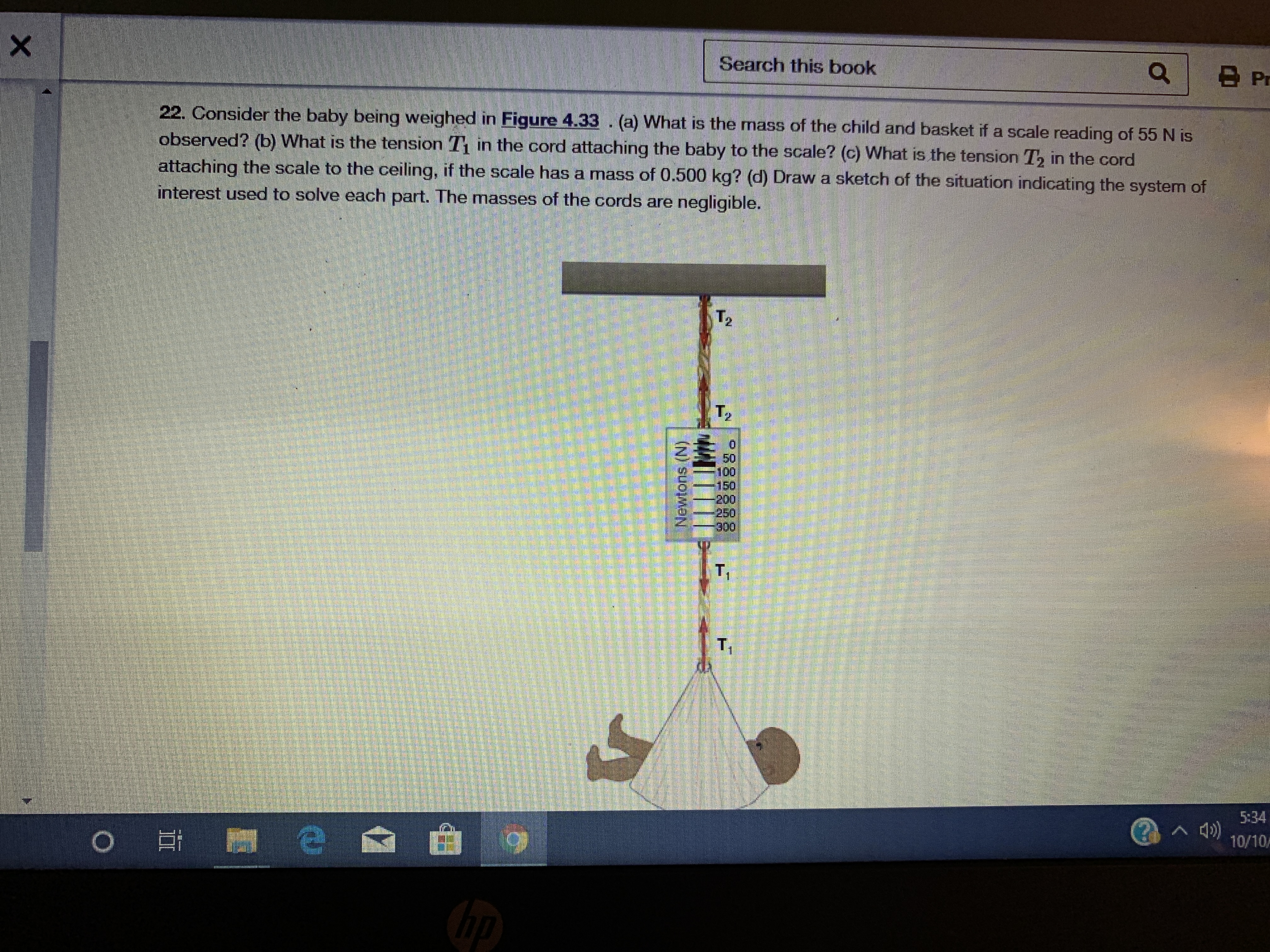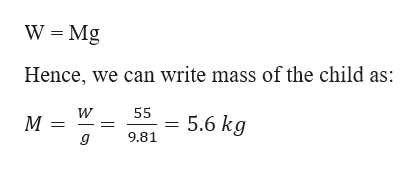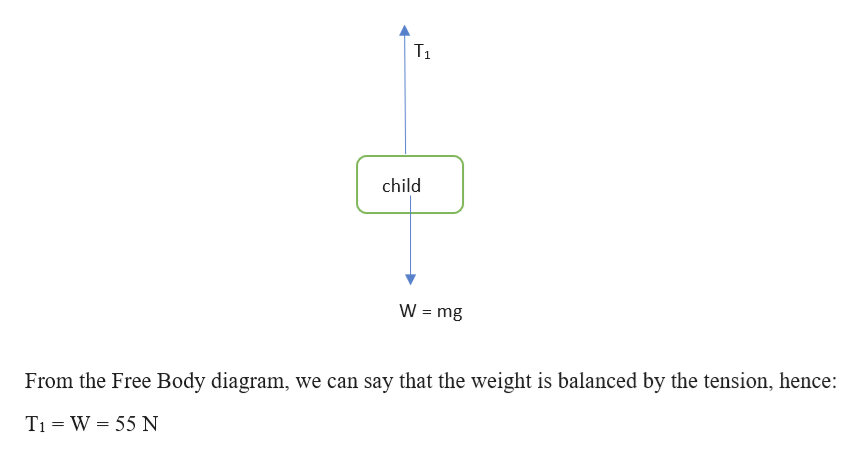B PrSearch this book22. Consider the baby being weighed in Figure 4.33. (a) What is the mass of the child and basket if a scale reading of 55 N isobserved? (b) What is the tension 1 in the cord attaching the baby to the scale? (c) What is the tension Th in the cordattaching the scale to the ceiling, if the scale has a mass of 0.500 kg? (d) Draw a sketch of the situation indicating the system ofinterest used to solve each part. The masses of the cords are negligible.T2T250100150200-250300T15:3410/10OXNewtons (N)

Question

What is the answer to this question?help_outlineImage TranscriptioncloseB Pr Search this book 22. Consider the baby being weighed in Figure 4.33. (a) What is the mass of the child and basket if a scale reading of 55 N is observed? (b) What is the tension 1 in the cord attaching the baby to the scale? (c) What is the tension Th in the cord attaching the scale to the ceiling, if the scale has a mass of 0.500 kg? (d) Draw a sketch of the situation indicating the system of interest used to solve each part. The masses of the cords are negligible. T2 T2 50 100 150 200 -250 300 T1 5:34 10/10 O X Newtons (N) fullscreen
Step 1

Given information:

Reading on the scale (W) = 55 N

Mass of the scale (Ms) = 0.500 kg

Step 2

Mass of the child and basket (M):

We know that weight is given as:help_outlineImage TranscriptioncloseW Mg Hence, we can write mass of the child as: 55 5.6kg 9.81 g fullscreen
Step 3

Part b:

Free body diagram to find th...help_outlineImage TranscriptioncloseT1 child W mg From the Free Body diagram, we can say that the weight is balanced by the tension, hence: TI W 55 N fullscreen

Want to see the full answer?

See Solution

Want to see this answer and more?

Our solutions are written by experts, many with advanced degrees, and available 24/7

See Solution
Tagged in

Physics